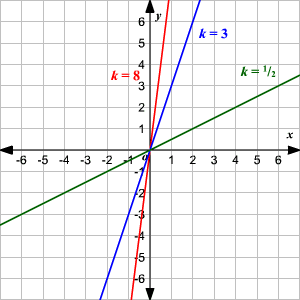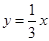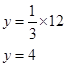# Proportional Relationships

A proportional relationship is one in which two quantities vary directly with each other. We say the variable $y$ varies directly as $x$ if:

$y=kx$

for some constant $k$ , called the constant of proportionality .

(Some textbooks describe a proportional relationship by saying that " $y$ varies proportionally with $x$ " or that " $y$ is directly proportional to $x$ .")

This means that as $x$ increases, $y$ increases and as $x$ decreases, $y$ decreases-and that the ratio between them always stays the same.

The graph of the proportional relationship equation is a straight line through the origin.Example 1:

Given that $y$ varies proportionally with  $x$ , with a constant of proportionality $k=\frac{1}{3}$ , find $y$ when $x=12$ .

Write the equation of the proportional relationship.

The variable $x$ varies proportionally with $y$ with a constant of proportionality equal to $\frac{1}{3}$ .

So,Substitute the given $x$ value.Example 2:

Given that $y$ varies proportionally with $x$ , find the constant of proportionality if $y=24$ and $x=3$ .

Write the equation of the proportional relationship.

$y=kx$

Substitute the given $x$ and $y$ values, and solve for $k$ .

$\begin{array}{l}24=k\cdot 3\\ k=8\end{array}$

Example 3:

Suppose $y$ varies proportionally with $x$ , and $y=30$ when $x=6$ . What is the value of $y$ when $x=100$ ?

Write the equation of the proportional relationship.

$y=kx$

Substitute the given $x$ and $y$ values, and solve for $k$ .

$30=k\cdot 6$

$k=5$

The equation is $y=5x$ . Now substitute $x=100$ and find $y$ .

$\begin{array}{l}y=5\cdot 100\\ y=500\end{array}$